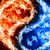0
Under review

# I want extend the scope of Oxyplot plotmodel for event handlers

Issak Sdu 5 years ago in API updated by Oystein Bjorke 5 years ago

I want that my plotview replies to more than one event handler, so I declared the plotmodel outside the event handler bloc, and the result was that it is out of scope of the event handler.

```using System; using System.Collections.Generic; using System.Drawing; using System.Windows.Forms; // for plotting/////// using OxyPlot; using OxyPlot.Series; namespace test {```

` `` ``public partial class MainForm : Form`

` `` ````{ ```` `` ````public MainForm() ```` `` ````{ ```` `` `` ````InitializeComponent(); ```` `` `` ``var myModel = new PlotModel { Title = "Example 1" };```` ```` `` `` ``plot1.Invalidate(true);```` ```` `` `` ``myModel.InvalidatePlot(true);```` ```` `` ````} `````` ```` `` ``void HScrollBar1Scroll(object sender, ScrollEventArgs e)`

``` ````{`

` ```` int hScrollBar1_Value = this.hScrollBar1.Value; Func<double,double> func1 = (x) => Math.Cos(x)/(1+hScrollBar1_Value); myModel.Series.Add(new FunctionSeries(func1, 0, 5, 0.1, "cos(x)")); plot1.Model = myModel; } } } ```
this way the vent handler has no effect and an error is generated. Is there any other way to make the event handlers access the plotmodel?Under review